## Example Questions

← Previous 1

### Example Question #1211 : Sat Mathematics

A sunglasses kiosk at the mall makes a $50 profit for every 6 pairs of sunglasses it sells. How many pairs of sunglasses must it sell to earn$1000 profit?

20

300

30

100

120

120

Explanation:

Divide the profit per 6 pairs into the total desired profit $1000/$50 = 20.

Multiply 20 by 6 sunglasses = 120 sunglasses.  Or use 6/50 = x/1000 and solve for x.

### Example Question #1 : How To Find Amount Of Profit

Ricky works at a shoe shop, and earns $40 in commission for each pair of shoes he sells plus a$100 weekly salary. If Ricky receives no other money, which of the following expressions represents the total dollar amount Ricky receives for a week in which he sells n shoes?

40(100 + n)

140 + n

140n

100 + 40n

100 + n

100 + 40n

Explanation:

If Ricky sells n shoes in a week, he earns $40n in commission. His salary is a constant$100 per week, so his total payout is $100 +$40n.

### Example Question #1 : Profit Margin

An entrepreneur started a company making floggles.  The factory requires $1000 worth of fixed expenses to keep it running every month. She is able to produce one floggle at the cost of$4 and sell one floggle at the cost of $6. If she produces and sells 500 floggles in one month, what is her profit? Possible Answers:$0

$2000$3000

$4000$1000

$0 Explanation: Profit = Income - Expenditures Income =$6/floggle times 500 floggles = $3000 Expenditures =$1000 + $4/floggle times 500 floggles =$1000 + $2000 =$3000

Profit = 3000 - 3000 = 0

### Example Question #331 : Arithmetic

You are planning a New Year’s Eve bash.  For each person attending, the caterer will charge you $15 for food,$10 for beverages, $5 for service. The band charges$2000 for the entire evening.  You also have to pay the venue $2500 to rent the location for the night and$3 for parking for each attendee.  If you expect 500 people to attend and you would like to make a $10000 profit for planning the event, how much must each ticket cost? Possible Answers:$62

$33$42

$53$60

$62 Explanation: First determine total cost. Caterer: Per person =$15 + $10 +$5 = $30 per person Parking: Per person =$3 per person

Total per person = $33$33 * 500 people = $16,500 Plus cost of renting venue + band =$2500 + $2000 =$4500

Total (net) cost =  $16,500 +$4500  = $21,000 Total (gross) cost = net cost + profit =$21,000 + $10,000 =$31,000

Cost per ticket = Gross cost / # of attendee = $31,000 / 500 =$62

### Example Question #1 : How To Find Amount Of Profit

The Widget Company has annual revenues of $150,000. Their expenses over the same time frame was$75,000.  What was the percent profit?

25%

60%

30%

50%

75%

50%

Explanation:

Profit = Revenue – Expense

% Profit = $Profit ÷$ Total Revenue

% Profit = ($150,000 –$75,000) ÷ $150,000 = 50% ### Example Question #1 : How To Find Amount Of Profit Nicki sold 20 albums at$5 each. How many albums should Minaj sell at $4.50 to earn more than Nicki? Possible Answers: 22 23 19 25 24 Correct answer: 23 Explanation: The answer is 23. 23*$4.50 = $103.50, which is more than what Nicki earned. ### Example Question #1 : Profit Margin During Laura and Anna’s bake sale, 35 brownies, 12 cupcakes and 23 glasses of lemonade were sold. These goods cost$44 for the raw ingredients, and they sold for $79. What is the average profit per item? Possible Answers:$0.35

$0.50$0.70

$0.25$1.75

$0.50 Explanation: Total profit ($35) divided by total items (70) yields the answer of $0.50 profit per item. ### Example Question #421 : Arithmetic Each wooden chair that a carpenter makes requires$20 worth of supplies. He then sells the chairs for $50 each. The carpenter recently discovered a new supplier that would allow him to spend 25% less on supplies. If he doesn't change his selling price, by what percent could the carpenter increase his profit by using the new supplier? Possible Answers: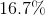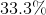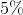Correct answer:Explanation: Using$20 worth of supplies and selling the chairs for $50 each, the carpenter is originally making a profit of$30 per chair.
The new supplier would reduce costs by 25% or 1/4. One-fourth of $20 is$5, so the new supplier would be $5 less, or$15.
If the selling price is the same ($50), then the carpenter would now make a profit of$35 per chair, a change of $5. To calculate percent increase, divide the actual change in profit by the original profit amount, and multiply the result by 100%: (Actual Change ÷ Original Amount) * 100% = 5/30 * 100% = 500%/30 = 16.7% ### Example Question #1 : How To Find Amount Of Profit A craftsman builds a cabinet. He pays$250 to buy the wood and miscellaneous materials for the cabinet.  He spends 20 hours building the cabinet.  If he values his time at $40 per hour and expects a profit margin of 50% above labor and materials, how much should he charge for the cabinet? Possible Answers: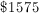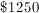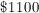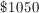Correct answer:Explanation: Total Cost = Material Cost + Labor Cost + Profit Labor Cost =$40/hour * 20 hours = $800 Profit Margin of 50% = Cost x 0.50 =$1050 x 0.50 = $525 Total Cost =$250 + $800 +$525 = $1575 ### Example Question #1 : How To Find Amount Of Profit The cost of manufacturing a single teddy bear is$6.25. A teddy bear company sells 200 bears for $1750. What is the profit percentage per single bear? Possible Answers: 28% 60% 72% 140% 40% Correct answer: 40% Explanation: First we must find out what the price is for one teddy bear, manufactured by this company. Thus we divide 1750 by 200 and find that each bear costs$8.75. To find out the profit per bear, we divide 8.75 by 6.25 to arrive at 1.4. The bears are thus sold for 140% of what it costs to make them, giving a 40% profit.